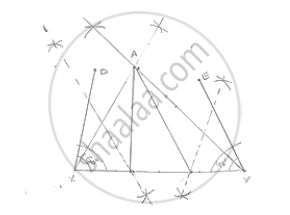# Construct a Triangle Whose Perimeter is 6.4 Cm, and Angles at the Base Are 60° and 45° . - Mathematics

Construct a triangle whose perimeter is 6.4 cm, and angles at the base are 60° and 45° .

#### SolutionSteps of construction:
1. Draw a line segment XY of 6.4cm.
2. Draw ∠DXY = B = 60° and ∠EYX =∠C = 45°
3. Draw the angle bisector of ∠DXY and ∠EYX which interest each other at A.
4. Draw the perpendicular bisector of AX and AY which interest XY at B and C respectively.
5. Join AB and AC
∴ Δ ABC is the required triangle.

Concept: Some Constructions of Triangles
Is there an error in this question or solution?

#### APPEARS IN

RD Sharma Mathematics for Class 9
Chapter 16 Constructions
Exercise 16.3 | Q 8 | Page 18

Share